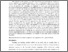# THE RESULT OF ASSESSMENT FOR STUDENTS IN SOLVING EXPONENTS AND LOGARITHMS PROBLEMS (CASE STUDY IN GRADE X CLASS MATHEMATICS AND NATURAL SCIENCE (MIA) 2 STATE SENIOR HIGH SCHOOL 1 DEPOK 2014/2015)

Fajar, Elmy Nuriyah (2014) THE RESULT OF ASSESSMENT FOR STUDENTS IN SOLVING EXPONENTS AND LOGARITHMS PROBLEMS (CASE STUDY IN GRADE X CLASS MATHEMATICS AND NATURAL SCIENCE (MIA) 2 STATE SENIOR HIGH SCHOOL 1 DEPOK 2014/2015). Seminar Nasional dan Temu Alumni "Peran Pendidikan dalam Pembangunan Karakter Bangsa". ISSN 978-602-96172-6-9Preview
Text
9.pdf

Download (406kB) | Preview
Official URL: https://pps.uny.ac.id

## Abstract

The objective of this research is to describe the result of assessment for students in solving exponents and logarithms problems in class X Mathematics and Natural Science (MIA) 2 Senior High School 1 Depok, Sleman included the rate of achievements level of solving the problem, the percentage of completion of each items, and the absorption percentage of students in exponents and logarithms material. In addition, the objective of this research is to describe the mistake that being done by students of class X Mathematics and Natural Science (MIA) 2 Senior High School 1 Depok, Sleman. This research is categorized into an explorative research with mixed-methods (quantitative and qualitative approach) with the participant-selection (qualitative-emphasized) model involving 32 students of class X Mathematics and Natural Science (MIA) 2 in Senior High School 1 Depok, Sleman. The 12 items of the instruments used are an essay on exponents and logarithms materials. The result of this research show that from the result of the essay test, the rate of achievements level of solving exponents and logarithms problem is about 54,59%, the percentage of completion of question 1a is 97%, question 1b is 79%, question 2a is 49%, question 2b is 41%, question 3a is 86%, question 3b is 55%, question 4 is 90%, question 5 is 53%, question 6 is 17%, question 7 is 74%, question 8 is 25%, and question 9 is 33%. The absorption percentage of students in exponents and logarithms materials is about 15,625%. Types of mistake that found on class X Mathematics and Natural Science (MIA) 2 Senior High School 1 Depok in solving Exponents and Logarithms problem are, 1) change the negative exponential form to the positive exponential form, 2) change the root form into the sum of the roots, 3) change the fraction exponential form into a root form and determine the result, 4) algebraic operations of root form, 5) rationalize the denominator of a fraction of root form, and 6) simplify the logarithms.

Item Type: Article Result of Assessment, Exponents and Logarithms Test, Types of Mistake Prosiding > ICERE 2014 Program Pascasarjana Eprints 13 Aug 2015 08:31 13 Aug 2015 08:31 http://eprints.uny.ac.id/id/eprint/24887

### Actions (login required)View Item